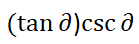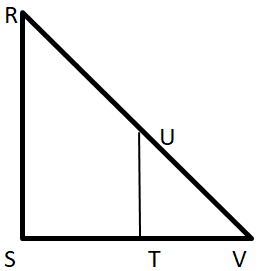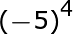# TExES Mathematics/Physical Science/Engineering 6-12 (274): Practice & Study Guide Final Exam

Free Practice Test Instructions:

Choose your answer to the question and click 'Continue' to see how you did. Then click 'Next Question' to answer the next question. When you have completed the free practice test, click 'View Results' to see your results. Good luck!

#### Question 1 1. Simplify the following trigonometric expression.#### Question 2 2. In right triangle RSV, segment RS is parallel to UT. If RS = 16, UT = 6.4 and TV = 4.8, determine the measure of SV.#### Question 12 12. Triangle ABC is a right triangle with Angle B as the right angle. If angle C has a measure of 40 degrees and AB = 7 units, determine the approximate measure of AC.#### Question 15 15. Solve the following expression: